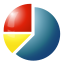⇦ | jfractionlab [main]
Last updated on: 2022-01-18 08:16 [UTC]

jfractionlab.desktop - 0.92-2 ⚙ all---
Type: desktop-application
ID: JFractionLab.desktop
Package: jfractionlab
Name:
C: JFractionLab
Summary:
C: Calculate Fractions is fun!
es: ¡Calcular con fracciones es divertido!
Description:
C: >-
<p>JFractionLab is a nice tool for math students that are learning the use and operation of fractions.</p>

<p>It provides a collection of nice exercices with a numeric and visual representation of the fractions.</p>

<p>JFractionLab shows the user every step of the calculation and therefore helps him to understand them.</p>

<p>It gives the user an answer after each input, making impossible to have a false answer.</p>
en: >-
<p>JFractionLab is a nice tool for math students that are learning the use and operation of fractions.</p>

<p>It provides a collection of nice exercices with a numeric and visual representation of the fractions.</p>

<p>JFractionLab shows the user every step of the calculation and therefore helps him to understand them.</p>

<p>It gives the user an answer after each input, making impossible to have a false answer.</p>
Categories:
- Education
- Math
Icon:
cached:
- name: jfractionlab_jfractionlab_icon.png
width: 64
height: 64
Launchable:
desktop-id:
- JFractionLab.desktop